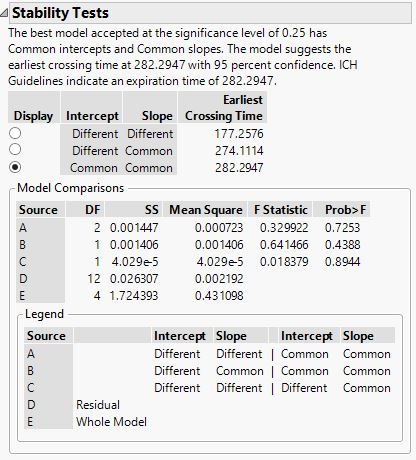Choose Language Hide Translation Bar
Highlighted

## Stability Test Model Comparisons - which P value to select for common slope AND intercepts?This is real basic question, but Help doesn't quite clear it up for me. In this case of common slope and common intercept. To express the p-value for the slope comparison, it would be the Source C p=0.8944.   Should I report the p-value for intercept comparison as Source B p=0.4388 or as Source A p=0.7523.

Thanks anyone!

1 ACCEPTED SOLUTION

Accepted Solutions
Highlighted

## Re: Stability Test Model Comparisons - which P value to select for common slope AND intercepts?

The order in the JMP report is the reverse of the order in the ICH guidance. That is, model comparison C is to reject common slope, model comparison B is to reject common intercept, and model comparison A is to reject both. So you are correct to use p-value for Source C for the slope evaluation and Source B for the intercept evaluation.

Learn it once, use it forever!
2 REPLIES 2
Highlighted

## Re: Stability Test Model Comparisons - which P value to select for common slope AND intercepts?

The order in the JMP report is the reverse of the order in the ICH guidance. That is, model comparison C is to reject common slope, model comparison B is to reject common intercept, and model comparison A is to reject both. So you are correct to use p-value for Source C for the slope evaluation and Source B for the intercept evaluation.

Learn it once, use it forever!
Highlighted

## Re: Stability Test Model Comparisons - which P value to select for common slope AND intercepts?

Mark

Thanks! Suggest the Help documentation get another example of common+common, and fail+fail, to show all the possibilities.

Steve
Article Labels

There are no labels assigned to this post.